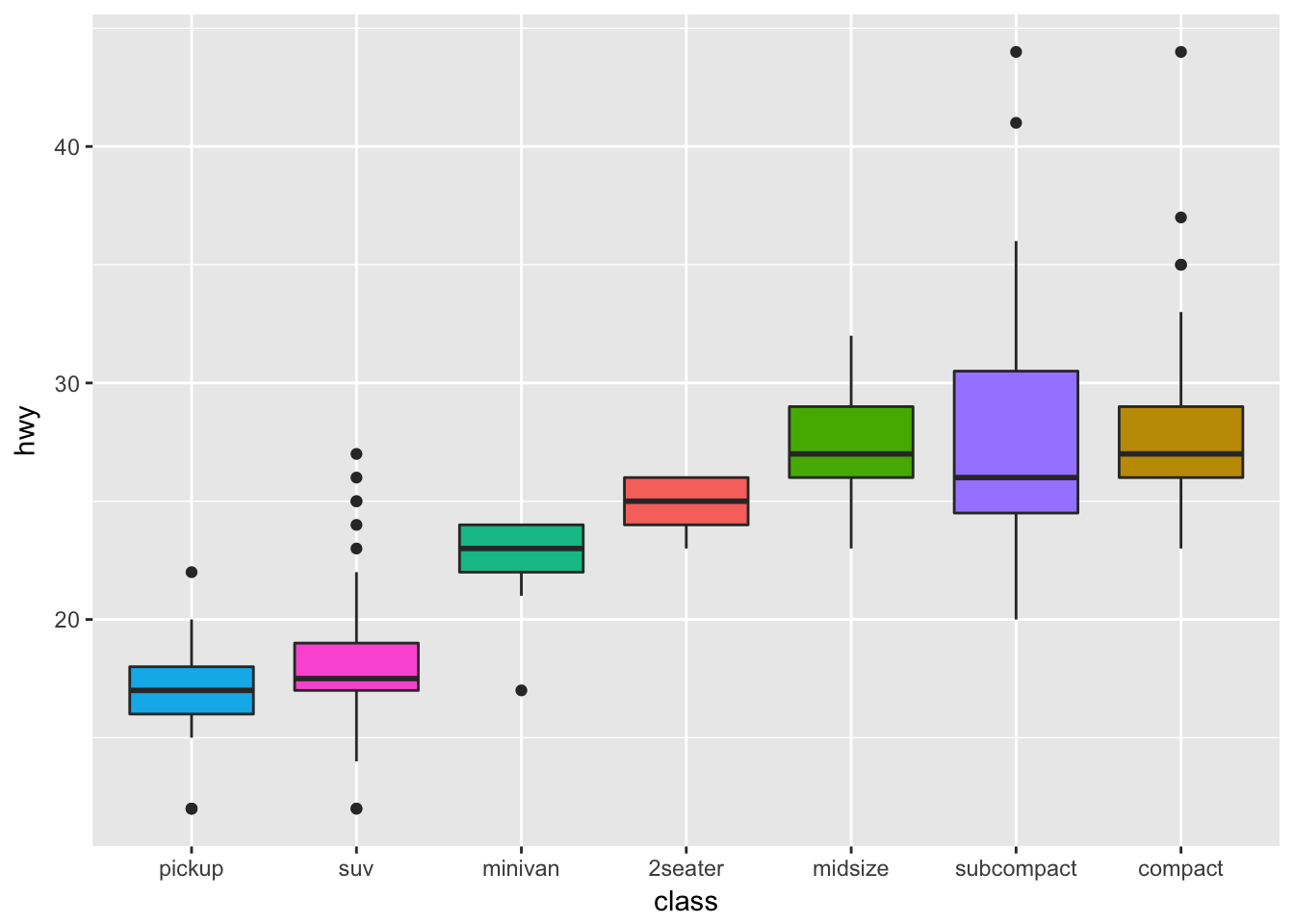Lab Goal: The simple goal of this lab is to get you thinking about R and RStudio again.

This lab can be completed with only R and without RStudio, but you should really use RStudio. For reference material, see Applied Statistics with R chapter two through six.

# Basic Calculations

[Exercise] Calculate $$e^2$$.

Solution:

exp(2)
##  7.389056

[Exercise] Calculate the natural log of 3.

Solution:

log(3)
##  1.098612

# Getting Help

y = c(0, 2, NA, 3, 4, 1, 9, 0)

[Exercise] Calculate the mean of y with the missing values removed. Use only the mean() function.

• Hint 1: Check the documentation for mean().
• Hint 2: If you’re really stuck, there always this approach.

Solution:

mean(y, na.rm = TRUE)
##  2.714286

# Packages

[Exercise] Run the following code:

ggplot(mpg, aes(x = reorder(class, hwy), y = hwy, fill = class)) +
geom_boxplot() +
xlab("class") +
theme(legend.position = "none")

To do so, you will need to make sure the ggplot2 package is installed, and loaded.

Solution:

# install.packages("ggplot2")
library(ggplot2)
ggplot(mpg, aes(x = reorder(class, hwy), y = hwy, fill = class)) +
geom_boxplot() +
xlab("class") +
theme(legend.position = "none")[Exercise] Modify the following code to run, but without loading the entire MASS library. The lda() function is from the MASS package.

lda(Species ~ ., data = iris)$means Solution: MASS::lda(Species ~ ., data = iris)$means
##            Sepal.Length Sepal.Width Petal.Length Petal.Width
## setosa            5.006       3.428        1.462       0.246
## versicolor        5.936       2.770        4.260       1.326
## virginica         6.588       2.974        5.552       2.026

# Vectors and Lists

x = 1:100

[Exercise] Calculate

$\sum_{i = 1}^{n} \ln(x_i).$

That is, sum the log of each element of x.

Solution:

sum(log(x))
##  363.7394

[Exercise] After running the following code, how many of the elements of some_vector are larger than 1? A good solution will use only one line of code.

set.seed(42)
some_vector = rnorm(100)

Solution:

sum(some_vector > 1)
##  17

# Probability

[Exercise] Consider a random variable $$X$$ that has a normal distribution with a mean of 5 and a variance of 9. Calculate

$P[X > c],$

for $$c = 1, 2, 3, 4, 5, 6, 7, 8, 9, 10.$$

Solution:

c = 1:10
pnorm(c, mean = 5, sd = 3, lower.tail = FALSE)
##   0.90878878 0.84134475 0.74750746 0.63055866 0.50000000 0.36944134
##   0.25249254 0.15865525 0.09121122 0.04779035